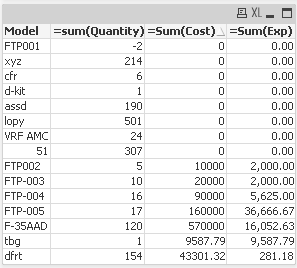# New to QlikView

Discussion board where members can get started with QlikView.

Announcements
Modernize Your QlikView Deployment webinar, Nov. 3rd. REGISTER
cancel
Showing results for
Did you mean:
HighlightedCreator

## How to remove Totals and other unwanted rows using Script?

Hi,

I have the attached file/table to load.

Have to calculate model wise Sum(Cost)/Sum(Qty) as Exp.

Also need to ensure that:

1. The totals are removed (highlighted in Red & Blue)

2. If a model is repeated, then the average of repeated rows has to be considered:

e.g in case of FTP-005  the Exp. would be 18333.34 i.e (100000/15) + (60000/2)) / 2

Nick

3 Replies
HighlightedMVP

Load your table this way to not consider the rows where you have Total on that row and make clean on the load script and then do calculation for Exp that you have and take to table itself.

Data:

FieldA,

[Field Center],

Model,

Quantity,

Cost

FROM

[Value by Sheet Aug17 - CSD.xlsx]

(ooxml, embedded labels, table is Sheet1)

Where right([Field Center],5) <> 'Total' and Right(FieldA,5) <> 'Total';

Left Join

Model,RowID,

Sum(Cost)/Sum(Quantity) as Exp

Resident Data Group By Model,RowID;HighlightedCreator

Hi Anand,

If a model is repeated, then the average of repeated rows has to be considered:

e.g in case of FTP-005  the Exp. would be 18333.34 i.e (100000/15) + (60000/2)) / 2

HighlightedMaster II

Hi nick

Please find the attached. Named after you.regards